Complex Numbers: Introduction (page 1 of 3)

Sections: Introduction, Operations with complexes, The Quadratic FormulaUp until now, you've been told that you can't take the square root of a negative number. That's because you had no numbers which were negative after you'd squared them (so you couldn't "go backwards" by taking the square root). Every number was positive after you squared it. So you couldn't very well square-root a negative and expect to come up with anything sensible.

Now, however, you can take the square root of a negative number, but it involves using a new number to do it. This new number was invented (discovered?) around the time of the Reformation. At that time, nobody believed that any "real world" use would be found for this new number, other than easing the computations involved in solving certain equations, so the new number was viewed as being a pretend number invented for convenience sake.

(But then, when you think about it, aren't all numbers inventions? It's not like numbers grow on trees! They live in our heads. We made them all up! Why not invent a new one, as long as it works okay with what we already have?)

Advertisement

Anyway, this new number was called "i", standing for "imaginary", because "everybody knew" that i wasn't "real". (That's why you couldn't take the square root of a negative number before: you only had "real" numbers; that is, numbers without the "i" in them.) The imaginary is defined to be: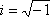Then: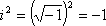Now, you may think you can do this: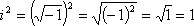But this doesn't make any sense! You already have two numbers that square to 1; namely –1 and +1. And i already squares to –1. So it's not reasonable that i would also square to 1. This points out an important detail: When dealing with imaginaries, you gain something (the ability to deal with negatives inside square roots), but you also lose something (some of the flexibility and convenient rules you used to have when dealing with square roots). In particular, YOU MUST ALWAYS DO THE i-PART FIRST!

• Simplify sqrt(–9).   Copyright © Elizabeth Stapel 2000-2011 All Rights Reserved
•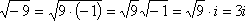(Warning: The step that goes through the third "equals" sign is "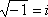", not "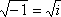". The i is outside the radical.)

• Simplify sqrt(–25).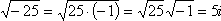• Simplify sqrt(–18).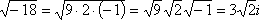• Simplify –sqrt(–6).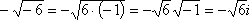In your computations, you will deal with i just as you would with x, except for the fact that x2 is just x2, but i2 is –1:

• Simplify 2i + 3i.

2i + 3i = (2 + 3)i = 5i

• Simplify 16i – 5i.

16i – 5i = (16 – 5)i = 11i

• Multiply and simplify (3i)(4i).

(3i)(4i) = (3·4)(i·i) = (12)(i2) = (12)(–1) = –12

• Multiply and simplify (i)(2i)(–3i).

(i)(2i)(–3i) = (2 · –3)(i · i · i) = (–6)(i2 · i)

=(–6)(–1 · i) = (–6)(–i) = 6i

Note this last problem. Within it, you can see that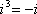, because i2 = –1. Continuing, we get: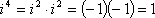This pattern of powers, signs, 1's, and i's is a cycle: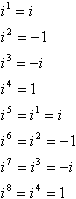In other words, to calculate any high power of i, you can convert it to a lower power by taking the closest multiple of 4 that's no bigger than the exponent and subtracting this multiple from the exponent. For example, a common trick question on tests is something along the lines of "Simplify i99", the idea being that you'll try to multiply i ninety-nine times and you'll run out of time, and the teachers will get a good giggle at your expense in the faculty lounge. Here's how the shortcut works:

i99 = i96+3 = i(4×24)+3 = i3 = –i

That is, i99 = i3, because you can just lop off the i96. (Ninety-six is a multiple of four, so i96 is just 1, which you can ignore.) In other words, you can divide the exponent by 4 (using long division), discard the answer, and use only the remainder. This will give you the part of the exponent that you care about. Here are a few more examples:

• Simplify i17.

i17 = i16 + 1 = i4 · 4 + 1 = i1 = i

• Simplify i120.

i120 = i4 · 30 = i4· 30 + 0 = i0= 1

• Simplify i64,002.

i64,002 = i64,000 + 2 = i4 · 16,000 + 2 = i2 = –1

Now you've seen how imaginaries work; it's time to move on to complex numbers. "Complex" numbers have two parts, a "real" part (being any "real" number that you're used to dealing with) and an "imaginary" part (being any number with an "i" in it). The "standard" format for complex numbers is "a + bi"; that is, real-part first and i-part last.

Top  |  1 | 2 | 3  |  Return to Index  Next >>

 Cite this article as: Stapel, Elizabeth. "Complex Numbers: Introduction." Purplemath. Available from     https://www.purplemath.com/modules/complex.htm. Accessed [Date] [Month] 2016

MathHelp.com Courses
 Copyright © 2021  Elizabeth Stapel   |   About   |   Terms of Use   |   Linking   |   Site Licensing Contact Us